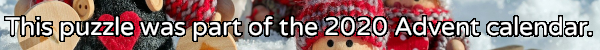mscroggs.co.uk
mscroggs.co.uksubscribe

# Puzzles

## 11 DecemberNoel has a large pile of cards. Half of them are red, the other half are black. Noel splits the cards into two piles: pile A and pile B.
Two thirds of the cards in pile A are red. Noel then moves 108 red cards from pile A to pile B. After this move, two thirds of the cards in pile B are red.
Note: There was a mistake in the original version of today's puzzle. The number 21 has been replaced with 108.

## 5 DecemberCarol rolled a large handful of six-sided dice. The total of all the numbers Carol got was 521. After some calculating, Carol worked out that the probability that of her total being 521 was the same as the probability that her total being 200. How many dice did Carol roll?

## Bending a straw

Two points along a drinking straw are picked at random. The straw is then bent at these points. What is the probability that the two ends meet up to make a triangle?

## The sixth cent

You toss 6 fair coins, and I toss 5 fair coins. What is the probability that you get more heads than I do?

## Marbles

A bag contains $$m$$ blue and $$n$$ yellow marbles. One marble is selected at random from the bag and its colour is noted. It is then returned to the bag along with $$k$$ other marbles of the same colour. A second marble is now selected at random from the bag. What is the probability that the second marble is blue?

## Fair dice

Timothy and Urban are playing a game with two six-sided dice. The dice are unusual: Rather than bearing a number, each face is painted either red or blue.
The two take turns throwing the dice. Timothy wins if the two top faces are the same color, and Urban wins if they're different. Their chances of winning are equal.
The first die has 5 red faces and 1 blue face. What are the colours on the second die?

## The blue-eyed sisters

If you happen to meet two of the Jones sister (two sisters chosen at random from all the Jones sisters), it is exactly an even-money bet that both will be blue-eyed. What is your best guess of the total number of Jones sisters?

## Equal opportunity

Can two (six-sided) dice be weighted so that the probability of each of the numbers 2, 3, ..., 12 is the same?

## Archive

Show me a random puzzle
▼ show ▼